# Latitude

﻿
Latitude
Longitude (λ) Latitude (φ)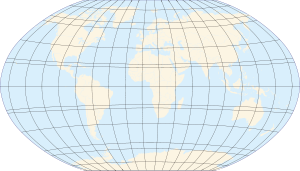Map of Earth Lines of longitude appear vertical with varying curvature in this projection, but are actually halves of great ellipses, with identical radii at a given latitude. Lines of latitude appear horizontal with varying curvature in this projection; but are actually circular with different radii. All locations with a given latitude are collectively referred to as a circle of latitude. The equator divides the planet into a Northern Hemisphere and a Southern Hemisphere, and has a latitude of 0°.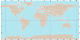See Geodesy topics. v · d · e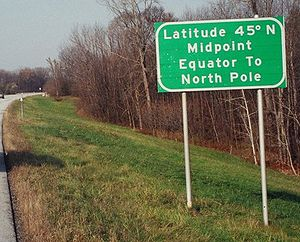Sign in northern Vermont (Since the earth isn't spherical, the halfway point equator to pole is actually 16 km north of latitude 45 deg.)

In geography, the latitude of a location on the Earth is the angular distance of that location south or north of the Equator. The latitude is an angle, and is usually measured in degrees (marked with °). The equator has a latitude of 0°, the North pole has a latitude of 90° north (written 90° N or +90°), and the South pole has a latitude of 90° south (written 90° S or −90°). Together, latitude and longitude can be used as a geographic coordinate system to specify any location on the globe.

Curves of constant latitude on the Earth (running east-west) are referred to as lines of latitude, or parallels. Each line of latitude is actually a circle on the Earth parallel to the equator, and for this reason lines of latitude are also known as circles of latitude. In spherical geometry, lines of latitude are examples of circles of a sphere, with the equator being a great circle.

Latitude (usually denoted by the Greek letter phi (φ)) is often measured in degrees, minutes and seconds. The Eiffel Tower has a latitude of 48° 51′ 29″ N-- that is, 48 degrees plus 51 minutes plus 29 seconds. Or latitude may be measured entirely in degrees, e.g. 48.85806° N.

If the Earth were actually spherical, and homogenous, and not rotating, then latitude at a point would just be the angle between a vertical line at that point and the plane of the equator. Everywhere on Earth a vertical line would point to the center of the Earth. In reality the earth is rotating and is not spherical, so a vertical line — a line in the direction of apparent gravity — doesn't point to the center of the Earth (except at the poles and the equator). If the Earth were homogenous, then a vertical line would still point to some point on the Earth's axis, and latitude at a point would still be the angle between the vertical line there and the plane of the equator.

But the Earth is not homogenous, and has mountains-- which have gravity and so can shift the vertical line away from the Earth's axis. The vertical line still intersects the plane of the equator at some angle; that angle is astronomical latitude, the latitude you would calculate from star observations. The latitude shown on maps and GPS devices is the angle between a not-quite-vertical line through the point and the plane of the equator; the not-quite-vertical line is perpendicular to the surface of the spheroid chosen to approximate the Earth's sea-level surface, rather than perpendicular to the sea-level surface itself.

## ExamplesDiagram showing the locations of the five major lines of latitude on an equirectangular projection of the Earth.

Besides the equator, four other lines of latitude are commonly used to mark maps of the Earth. Each of these lines plays an important role in the geometrical relationship between the Earth and the Sun:

Only at latitudes in the tropics is it possible for the sun to be directly overhead (at the zenith). Alternatively, only within the Arctic and Antarctic circles is it possible to have a polar night or midnight sun.

The latitudes of the two tropics are determined by the axial tilt of the Earth with respect to the sun, which is approximately 23° 26′ 21″. The latitudes of the Arctic and Antarctic circles are the complements to this angle.

### Table of latitudes

The following table lists the regions of the Earth (excluding oceans) at various latitudes:

Latitude Locations
90° N North pole
75° N Arctic ocean; northern Siberia, Russia; northern Canada; Greenland
60° N Shetland Islands (United Kingdom); Norway (through Oslo); Sweden; Finland; Russia (through St. Petersburg); Alaska; Yukon to north tip of Newfoundland in Canada; south tip of Greenland
45° N France; Italy; Croatia; Bosnia; Serbia; Romania; the Black sea; Ukraine; Russia; the Caspian sea; Kazakhstan; Xinjiang (China); Mongolia; Inner Mongolia and Manchuria (China); far east Russia; Hokkaido (Japan); Kurils (Russia); Oregon to Michigan in the United States; Lake Huron; Ontario, Canada; Maine (USA); Nova Scotia (Canada)
30° N Morocco; Algeria; Libya; Egypt; Jordan; Saudi Arabia; Iraq; Kuwait; the Persian Gulf; Iran; Afghanistan; Pakistan; India; Nepal; southern China; south tip of Japan; northern Mexico; Texas, Louisiana and Florida (USA); Savage Islands (Portugal)
15° N Senegal; Mauritania; Mali; Burkina Faso; Niger; Chad; Sudan; Eritrea; the Red sea; Yemen; India; Myanmar; Thailand; Laos; Vietnam; Philippines; Northern Mariana Islands; southern Mexico; Guatemala; Honduras; Nicaragua; Caribbean Islands (between Dominica and Martinique); Cape Verde
São Tomé and Príncipe; Gabon; Republic of the Congo; Democratic Republic of the Congo; Uganda; Lake Victoria; Kenya; Somalia; Maldive Islands; Indonesia; Kiribati; Galápagos Islands and mainland Ecuador; Colombia; northern Brazil
15° S Angola; Zambia; Mozambique; Malawi; Madagascar; northern Australia; Vanuatu; French Polynesia; Peru; Bolivia; Brazil
30° S South Africa; Lesotho; southern Australia; Kermadec Islands; Chile; Argentina; southern Brazil
45° S New Zealand; Chile; Argentina
60° S entirely ocean (slightly north of the South Orkney Islands); sometimes considered the northern boundary of the Southern ocean
75° S Dome C, Antarctica
90° S South pole

## Subdivisions

A degree is divided into 60 minutes. One minute can be further divided into 60 seconds. An example of a latitude specified in this way is 13°19'43″ N (for greater precision, a decimal fraction can be added to the seconds). An alternative representation uses only degrees and minutes, where the seconds are expressed as a decimal fraction of minutes: the above example would be expressed as 13°19.717' N. Degrees can also be expressed singularly, with both the minutes and seconds incorporated as a decimal number and rounded as desired (decimal degree notation): 13.32861° N. Sometimes, the north/south suffix is replaced by a negative sign for south (−90° for the South Pole).

## Effect of latitudeAverage temperatures vary strongly with latitude.

A region's latitude has a great effect on its climate and weather. Latitude more loosely determines prevailing winds, and other physical characteristics of geographic locations. Auroral activity is common at high geomagnetic latitudes.

Some observers have noted a correlation between the latitude of a country and its economic activity; see North-South divide.

## Elliptic parameters

Because most planets (including Earth) are ellipsoids of revolution, or spheroids, rather than spheres, both the radius and the length of arc varies with latitude. This variation requires the introduction of elliptic parameters based on an ellipse's angular eccentricity,$\alpha\,\!$ (which equals$\arccos\left(\frac{b}{a}\right)\,\!$, where$a\;\!$ and$b\;\!$ are the equatorial radius (6,378,137.0 m for Earth) and the polar radius (6,356,752.3142 m for Earth), respectively;$\sin^2(\alpha)\,\!$ is the first eccentricity squared,${e^2}\,\!$; and$2\sin^2\left(\frac{\alpha}{2}\right)\;\!$ or$1-\cos(\alpha)\,\!$ is the flattening,${f}\,\!$. Utilized in creating the integrands for curvature is the inverse of the principal elliptic integrand,$E'\,\!$:$n'(\phi)=\frac{1}{E'(\phi)} =\frac{1}{\sqrt{1-(\sin(\phi)\sin(\alpha))^2}};\,\!$\begin{align} M(\phi)&=a\cdot\cos^2(\alpha)n'^3(\phi) =\frac{(ab)^2}{\Big((a\cos(\phi))^2+(b\sin(\phi))^2\Big)^{3/2}};\\ N(\phi)&=a{\cdot}n'(\phi) =\frac{a^2}{\sqrt{(a\cos(\phi))^2+(b\sin(\phi))^2}}.\end{align}\,\!

## Degree length

On the Earth's surface the length of an arcdegree of north–south latitude difference is about 60 nautical miles, 111 kilometres or 69 statute miles at any latitude. The length of an arcdegree of east-west longitude difference is about the same at the equator as the north-south, reducing to zero at the poles.

If the Earth were spherical a degree of latitude would cover exactly 60 nautical miles anywhere from the equator to the poles. In fact the Earth is slightly squashed — its "diameter" from pole to pole is slightly less than its equatorial diameter — making a north-south line on the Earth's surface more sharply curved near the equator than near the poles. Which means at the equator a degree of latitude covers 1% less north-south distance than it does at the poles.

On the WGS84 spheroid, the length in meters of a degree of latitude at latitude φ (that is, the distance along a north-south line from latitude (φ - 0.5) degrees to (φ + 0.5) degrees) is about

111132.954 - 559.822(cos 2φ) + 1.175(cos 4φ)

(Those coefficients can be improved, but as they stand the distance they give is correct within a centimeter.)

of curvature$M \;\!$
Surface distance
per 1° change
in latitude
of curvature$N \;\!$
Surface distance
per 1° change
in longitude
6335.44 km 110.574 km 6378.14 km 111.320 km
15° 6339.70 km 110.649 km 6379.57 km 107.551 km
30° 6351.38 km 110.852 km 6383.48 km 96.486 km
45° 6367.38 km 111.132 km 6388.84 km 78.847 km
60° 6383.45 km 111.412 km 6394.21 km 55.800 km
75° 6395.26 km 111.618 km 6398.15 km 28.902 km
90° 6399.59 km 111.694 km 6399.59 km 0.000 km

## Auxiliary latitudes

There are six auxiliary latitudes that have applications to special problems in geodesy, geophysics and the theory of map projections:

• geocentric latitude,
• reduced (or parametric) latitude,
• authalic latitude,
• rectifying latitude,
• conformal latitude,
• isometric latitude.

When the word 'latitude' is used without qualification it is to be taken as the geodetic latitude, sometimes called the geographic latitude.

All the auxiliary latitudes, except the isometric latitude, are numerically close to the geodetic latitude, the difference being zero at the poles and on the equator and attaining a maximum, between 5 and 10 minutes of arc, at a geodetic latitude a few arc minutes from 45 degrees. The geodetic latitude is greater than (or equal to) all auxiliary latitudes.

In the theory of map projections the auxiliary latitudes are typically employed as intermediate constructs in a transformation from geodetic coordinates to Eastings and Northings in the projection plane. The end user has no need of a numerical value such as the authalic latitude of the Eiffel tower.

The auxiliary latitudes defined below have been expressed in terms of the semi-major axis, a, and the eccentricity, e, in forms which agree, apart from notational variants, with those given in the standard reference for map projections, namely Map projections — a working manual by Snyder. Derivations which assume some knowledge of the geometrical properties of the ellipsoid may be found in Adams. Pedagogical discussions of the geometry of the ellipsoid may be found in Rapp (Chapter 3) and Osborne (Chapters 5,6)

### Geocentric latitude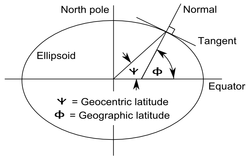The definition of geodetic (or geographic) and geocentric latitudes.

The geocentric latitude, denoted here by ψ, is the angle between the equatorial plane and the radius from the centre to a point on the surface. The relation between the geodetic latitude (ϕ) and the geocentric latitude (ψ) is$\psi(\phi)=\arctan\left[(1-e^2)\tan\phi\right]\;\!.$

The geodetic and geocentric latitudes are equal at the equator and poles. The value of the squared eccentricity is approximately 0.007 (depending on the choice of ellipsoid) and the maximum difference of (ϕ − ψ) is approximately 11.5 minutes of arc at a geodetic latitude of 45°6′.

### Reduced (or parametric) latitudeDefinition of the reduced latitude (β) on the ellipsoid.

The reduced or parametric latitude, β, is defined by the radius drawn from the centre of the ellipsoid to that point on the surrounding sphere (of radius a) which is the vertical projection (parallel to Earth's axis of rotation) of a point on the ellipsoid at latitude$\scriptstyle\phi$. It was so-called by Bessel who solved problems for geodesics on the ellipsoid by reducing them to the equivalent problem on the sphere. It is widely used in the theory of projections. In terms of the geodetic latitude$\beta(\phi)=\arctan\left[\sqrt{1-e^2}\tan\phi\right]\,\!$

The alternative name arises from the parameterization of the equation of the ellipse describing a meridian section. In terms of Cartesian coordinates p, the distance from the axis, and z, the distance above the equatorial plane, the equation of the ellipse is$\frac{p^2}{a^2} + \frac{z^2}{b^2} =1 .$

The Cartesian coordinates of the point are parameterized by$p=a\cos\beta, \qquad z=b\sin\beta.$

### Authalic latitude

The authalic latitude, ξ, gives an area-preserving transformation to a sphere.$\xi(\phi)=\arcsin\left(\frac{q(\phi)}{q_p}\right)\,\!$

where$q(\phi)= \frac{(1 - e^2)\sin\phi}{1 - e^2 \sin^2 \phi} -\frac{1-e^2}{2e}\ln \left(\frac{1-e\sin\phi}{1+e\sin\phi}\right),$

and$q_p = q(\pi/2) =1-\frac{1-e^2}{2e}\ln \left(\frac{1-e}{1+e}\right),\,$

and the radius of the sphere is taken as$R_q=a\sqrt{q_p/2}.\,$

Using the authalic latitude as a latitude on a sphere of the above radius defines an equal area mapping from the ellipsoid to the sphere. If the sphere is then projected to the plane with an area projection the result is an area-preserving double projection from the ellipsoid to the plane.

### Rectifying latitude

The rectifying latitude, μ, is the meridian distance scaled so that its value at the poles μ is equal 90 degrees or π/2 radians:$\mu(\phi)=\frac{\pi}{2}\frac{m(\phi)}{m_p}\,\!$

where the meridian distance from the equator to a latitude φ is (see Meridian arc)$m(\phi)= a(1 - e^2)\int_0^\phi \left (1 - e^2 \sin^2 \phi \right )^{-3/2} d\phi,$

and the length of the meridian quadrant to the pole is$m_p = m(\pi/2).\,$

Using the rectifying latitude to define a latitude on a sphere of radius$R=\frac{2m_p}{\pi}$

defines a projection from the ellipsoid to the sphere such that all meridians have true length and uniform scale. The sphere may then be projected to the plane with an equirectangular projection to give a double projection from the ellipsoid to the plane such that all meridians have true length and uniform meridian scale.

### Conformal latitude

The conformal latitude, χ, gives an angle-preserving (conformal) transformation to the sphere.$\chi(\phi)=2\arctan\left[ \left(\frac{1+\sin\phi}{1-\sin\phi}\right) \left(\frac{1-e\sin\phi}{1+e\sin\phi}\right)^{\!\textit{e}} \;\right]^{1/2} -\frac{\pi}{2} \;\!$.

This expression is sometimes given in the form$\chi(\phi)=2\arctan\left[ \tan\left(\frac{\phi}{2}+\frac{\pi}{4}\right) \left(\frac{1-e\sin\phi}{1+e\sin\phi}\right)^{\!\textit{e}/2} \;\right] -\frac{\pi}{2} \;\!$.

This kind of latitude defines a transformation from the ellipsoid to a sphere of arbitrary radius such that the angle of intersection between any two lines on the ellipsoid is the same as the corresponding angle on the sphere (so that the shape of small elements is well preserved). A further conformal transformation from the sphere to the plane gives a conformal double projection from the ellipsoid to the plane. This is not the only way of generating such a conformal projection. For example, the 'exact' version of the Transverse Mercator projection on the ellipsoid is not a double projection. (It does, however, involve a generalisation of the conformal latitude to the complex plane).

### Isometric latitude

The isometric latitude, ψ (not to be confused with the geocentric latitude notation), is very important in the development of the ellipsoidal versions of the normal Mercator projection and the Transverse Mercator projection. The name "isometric" arises from the fact that at any point on the ellipsoid equal increments of ψ and longitude λ give rise to equal distance displacements along the meridians and parallels respectively. The graticule defined by the lines of constant ψ and constant λ, divides the surface of the ellipsoid into a mesh of squares (of varying size). The isometric latitude is zero at the equator but rapidly diverges from the geodetic latitude, tending to infinity at the poles. The conventional notation is given in Snyder:15:$\psi(\phi) =\ln\left[\tan\left( \frac{\pi}{4}+\frac{\phi}{2}\right) \right] + \frac{e}{2}\ln\left[ \frac{1-e\sin\phi}{1+e\sin\phi} \right]$

For the normal Mercator projection (on the ellipsoid) this function defines the spacing of the parallels: if the length of the equator on the projection is E (units of length or pixels) then the distance, y, of a parallel of latitude φ from the equator is$y(\phi)=\frac{E}{2\pi}\psi(\phi).$

### Comparison of selected types

The following plot shows the differences between the types of latitude. The data used are found in the table following the plot. Please note that the values in the table are in minutes, not degrees, and the plot reflects this as well. Also observe that the conformal symbols are hidden behind the geocentric due to being very close in value. Finally it is important to mention also that these differences don't mean that the use of one specific latitude will necessarily cause more distortions than the other (the real fact is that each latitude type is optimized for achieving a different goal).Approximate difference from geographic latitude ("Lat")
Lat$\phi\,\!$
Reduced$\phi-\beta\,\!$
Authalic$\phi-\xi\,\!$
Rectifying$\phi-\mu\,\!$
Conformal$\phi-\chi\,\!$
Geocentric$\phi-\psi\,\!$
0.00′ 0.00′ 0.00′ 0.00′ 0.00′
1.01′ 1.35′ 1.52′ 2.02′ 2.02′
10° 1.99′ 2.66′ 2.99′ 3.98′ 3.98′
15° 2.91′ 3.89′ 4.37′ 5.82′ 5.82′
20° 3.75′ 5.00′ 5.62′ 7.48′ 7.48′
25° 4.47′ 5.96′ 6.70′ 8.92′ 8.92′
30° 5.05′ 6.73′ 7.57′ 10.09′ 10.09′
35° 5.48′ 7.31′ 8.22′ 10.95′ 10.96′
40° 5.75′ 7.66′ 8.62′ 11.48′ 11.49′
45° 5.84′ 7.78′ 8.76′ 11.67′ 11.67′
50° 5.75′ 7.67′ 8.63′ 11.50′ 11.50′
55° 5.49′ 7.32′ 8.23′ 10.97′ 10.98′
60° 5.06′ 6.75′ 7.59′ 10.12′ 10.13′
65° 4.48′ 5.97′ 6.72′ 8.95′ 8.96′
70° 3.76′ 5.01′ 5.64′ 7.52′ 7.52′
75° 2.92′ 3.90′ 4.39′ 5.85′ 5.85′
80° 2.00′ 2.67′ 3.00′ 4.00′ 4.01′
85° 1.02′ 1.35′ 1.52′ 2.03′ 2.03′
90° 0.00′ 0.00′ 0.00′ 0.00′ 0.00′

## Corrections for altitude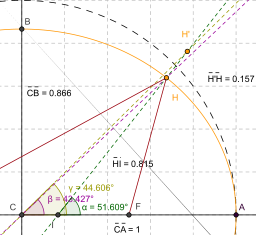Line IH is normal to the spheroid representing the Earth (colored orange) at point H. The angle it forms with the equator (represented by line CA) corresponds to the point's geodetic latitude.

When converting from geodetic ("common") latitude to other types of latitude, corrections must be made for altitude for systems which do not measure the angle from the normal of the spheroid. For example, in the figure at right, point H (located on the surface of the spheroid) and point H' (located at some greater elevation) have different geocentric latitudes (angles β and γ respectively), even though they share the same geodetic latitude (angle α). Note that the flatness of the spheroid and elevation of point H' in the image is significantly greater than what is found on the Earth, exaggerating the errors inherent in such calculations if left uncorrected. Note also that the reference ellipsoid used in the geodetic system is itself just an approximation of the true geoid, and therefore introduces its own errors, though the differences are less severe. (See Astronomical latitude, below.)

## Astronomical latitude

A fundamentally different measure of latitude is the astronomical latitude, which is the angle between the equatorial plane and the normal to the geoid (i.e. a plumb line). This is the only "latitude" that is not based on the spheroid being used to approximate the Earth's surface. It differs from the geodetic latitude only slightly (usually not more than a few thousandths of a degree) due to the slight deviations of the geoid from the reference ellipsoid.

Astronomical latitude is not to be confused with declination, the coordinate astronomers use to describe the locations of stars north/south of the celestial equator (see equatorial coordinates), nor with ecliptic latitude, the coordinate that astronomers use to describe the locations of stars and solar system bodies north/south of the ecliptic (see ecliptic coordinates).

## Paleolatitude

Continents move over time, due to continental drift, taking whatever fossils and other features of interest they may have with them. Particularly when discussing fossils, it is often more useful to know where the fossil was when it was laid down, than where it is when it was dug up: this is called the paleolatitude of the fossil. The paleolatitude can be constrained by paleomagnetic data. If tiny magnetisable grains are present when the rock is being formed, these will align themselves with Earth's magnetic field like compass needles. A magnetometer can deduce the orientation of these grains by subjecting a sample to a magnetic field, and the magnetic declination of the grains can be used to infer the latitude of deposition.

## Footnotes

1. ^ a b Snyder, John P. (1987). Map Projections – A Working Manual. U.S. Geological Survey Professional Paper 1395. United States Government Printing Office, Washington, D.C..
2. ^ Adams, Oscar S (1921). Latitude Developments Connected With Geodesy and Cartography, (with tables, including a table for Lambert equal area meridional projection). Special Publication No. 67 of the US Coast and Geodetic Survey. Warning: Adams uses the nomenclature isometric latitude for the conformal latitude of this article.
3. ^ Rapp, Richard H. (1991). Geometric Geodesy, Part I, Dept. of Geodetic Science and Surveying, Ohio State Univ., Columbus, Ohio.
4. ^ Osborne, P (2008)The Mercator Projections.

Wikimedia Foundation. 2010.

Synonyms:

### Look at other dictionaries:

• latitude — [ latityd ] n. f. • 1314; lat. latitudo « largeur » I ♦ 1 ♦ Vx Largeur. Spécialt Large acception ou extension. 2 ♦ (XVIe) Fig. Faculté, pouvoir d agir (en toute liberté). Mod. Donner, laisser toute latitude à qqn pour faire qqch. ⇒ …   Encyclopédie Universelle

• latitude — lat‧i‧tude [ˈlættjuːd ǁ tuːd] noun [uncountable] JOURNALISM freedom to choose what you do or say: latitude in • The new guidelines give banks more latitude in making loans. • States generally have wide latitude in setting tax policies. * * *… …   Financial and business terms

• Latitude — Lat i*tude, n. [F. latitude, L. latitudo, fr. latus broad, wide, for older stlatus; perh. akin to E. strew.] 1. Extent from side to side, or distance sidewise from a given point or line; breadth; width. [1913 Webster] Provided the length do not… …   The Collaborative International Dictionary of English

• latitude — LATITUDE. subst. f. Terme d Astronomie & de Geographie. La distance de l Equateur aux Poles. Latitude boreale. latitude australe. Paris est à tant de degrez de latitude. prendre les latitudes. le degré de latitude …   Dictionnaire de l'Académie française

• Latitude — (Кейптаун,Южно Африканская Республика) Категория отеля: Адрес: 301 Latitude, Corner Cavalc …   Каталог отелей

• Latitude ON — (promoted with a capitalized ON ) is a lightweight combination of software and hardware [http://www.pocket lint.co.uk/news/news.phtml/16829/17853/dell latitude on email boot.phtml] http://www.dell.com/content/topics/global.aspx/corp/pressoffice/en… …   Wikipedia

• latitude — [lat′ə to͞od΄, lat′ətyo͞od΄] n. [OFr < L latitudo < latus, wide: see LATERAL] 1. Rare breadth; width 2. Rare extent; scope; range of applicability 3. freedom from narrow restrictions; freedom of opinion, conduct, or action 4. see… …   English World dictionary

• Latitude 37 — (Окленд,Новая Зеландия) Категория отеля: 4 звездочный отель Адрес: 20 Pakenham Street Ea …   Каталог отелей

• Latitude — ist die Bezeichnung für: die Geographische Breite eine Modellreihe von Notebook Computern des Herstellers Dell den Renault Latitude einen Service zur Ortung von Mobiltelefonen in Google Maps …   Deutsch Wikipedia

• latitude — late 14c., breadth, from O.Fr. latitude (13c.) and directly from L. latitudo breadth, width, extent, size, from latus wide, from PIE root *stele to spread (Cf. O.C.S. steljo to spread out, Armenian lain broad ). Geographical sense also is from… …   Etymology dictionary

• Latitüde — (franz., lat. latitudo), Breite (besonders geographische), Weite, namentlich Spielraum, Freiheit der Bewegung; latitudinal, auf Breite (Breitengrad) bezüglich …   Meyers Großes Konversations-Lexikon